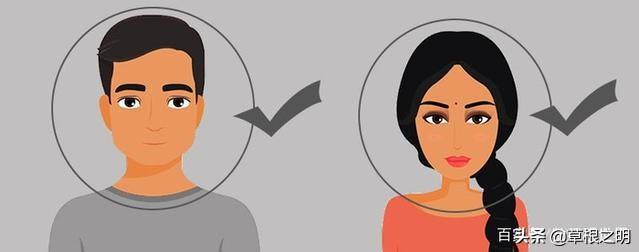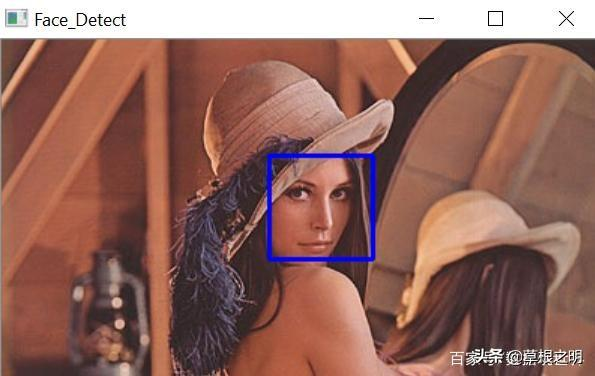人脸识别属于人工智能吗(AI算法如何识别人脸脸型)OpenCV：是一个主要针对实时计算机视觉的编程函数库。本文旨在使用OpenCV和Python/C++从图像中检测面部。Python

import numpy as np

import cv2 as cv

gray = cv.cvtColor(img, cv.COLOR_BGR2GRAY)

scaleFactor：在前后两次相继的扫描中，搜索窗口的比例系数。例如1.3指将搜索窗口依次扩大30%。

minNeighbors：构成检测目标的相邻矩形的最小个数。如果组成检测目标的小矩形的个数和小于minNeighbors都会被排除。

for (x,y,w,h) in faces:

cv.rectangle(img,(x,y),(x+w,y+h),(255,0,0),2)

roi_gray = gray[y:y+h, x:x+w]

roi_color = img[y:y+h, x:x+w]

cv.imshow(\’Face_Detect\’,img)

cv.waitKey(0)

cv.destroyAllWindows()